### Day 12 - Limit Problems - 09.03.14

 Bell Ringer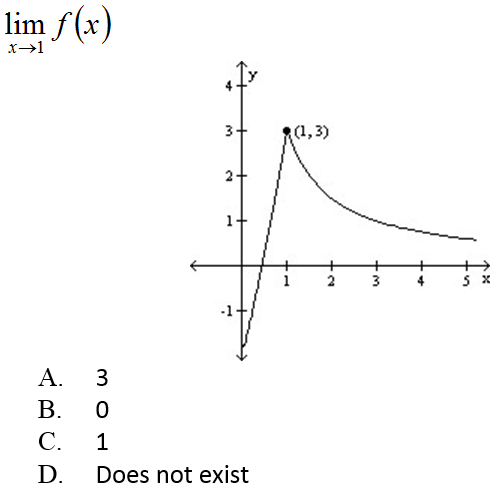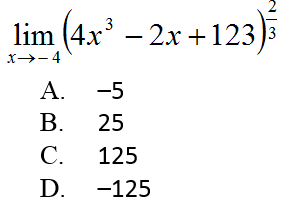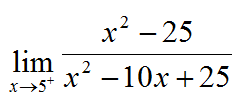infinitynegative infinity0 / 00none of the above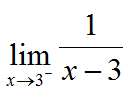infinitynegative infinity03none of the above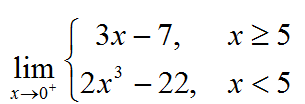-7-22-4-20none of the aboveReviewFinding limits using a tableFinding limits using a graphEpsilon-Delta Limit ProofsLimit PropertiesTrig FunctionsDividing Out/Rationalizing TechniquesFunctions that agree at all but one pointOne-sided LimitsInfinite LimitsPrecalculusDomain of a FunctionAsymptotesLessonGo over problems from yesterdayFind the values of the constants a and b such that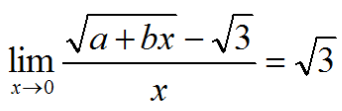Consider the function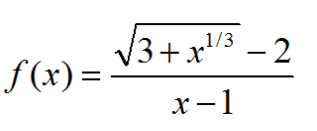Find the domain of f.Calculate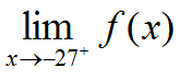Calculate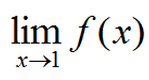Determine all values of the constant a such that the following function is continuous for all real numbers.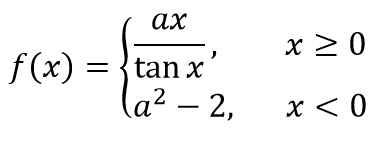Let a be a nonzero constant. Prove that if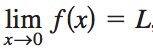, thenShow by means of an example that a must be nonzero.Create student lesson for tomorrow's student-led teachingStart student lessonsExit TicketComplete previous day's Exit Ticket if you have not already done so. Lesson Objective(s) How are the concepts in chapter 1 related and applied? Standard(s) APC.2 Define and apply the properties of limits of functions. Limits will be evaluated graphically and algebraically. Includes: ​limits of a constant ​limits of a sum, product, and quotient ​one-sided limits ​limits at infinity, infinite limits, and non-existent limits*APC.3Use limits to define continuity and determine where a function is continuous or discontinuous.Includes:​continuity in terms of limitscontinuity at a point and over a closed interval​application of the Intermediate Value Theorem and the Extreme Value Theorem​geometric understanding and interpretation of continuity and discontinuityAPC.4Investigate asymptotic and unbounded behavior in functions.Includes:describing and understanding asymptotes in terms of graphical behavior and limits involving infinity